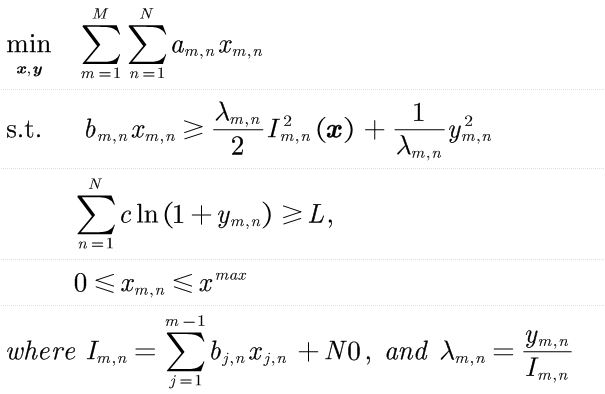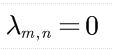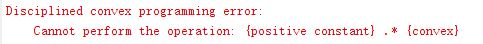# What's wrong with my codes? Cannot perform the operation: {positive constant} .* {convex}

The following figure is the optimization problem, and when lambda_{m,n}=0, the error message pops up. Could anyone help me please? Thanks in advance.where a,b,c, L and N0 are constants.
And my code is as following:

If, the error message pops up:inv_pos(0)
ans =
Inf

which is in accordance with the help

help inv_pos
inv_pos Reciprocal of a positive quantity.
inv_pos(X) returns 1./X if X is positive, and +Inf otherwise.
X must be real.

`````` For matrices and N-D arrays, the function is applied to each element.

Disciplined convex programming information:
inv_pos is convex and nonincreasing; therefore, when used in CVX
specifications, its argument must be concave (or affine).
``````

CVX doesn’t like `Inf` as input data in CVX expressions.

Thanks, Mark. But it still reports the same error even if I have added an EPS=2.2204e-16 to the value of lam. So how can I deal with it?

Maybe that input data doesn’t make sense. Or the form of the model needs to be changed for such extreme input data, if in fact such input data makes any sense at all.

it is the model author/your problem, not mine, so I have no idea what makes sense, or even any idea what the purpose of the model is.

Thank you, Mark, thank you for your patience. And I’ll check my model again.